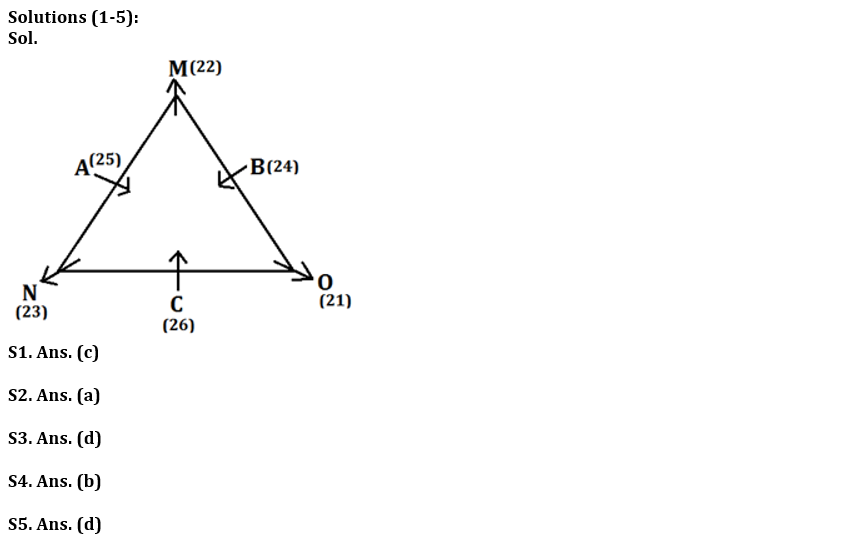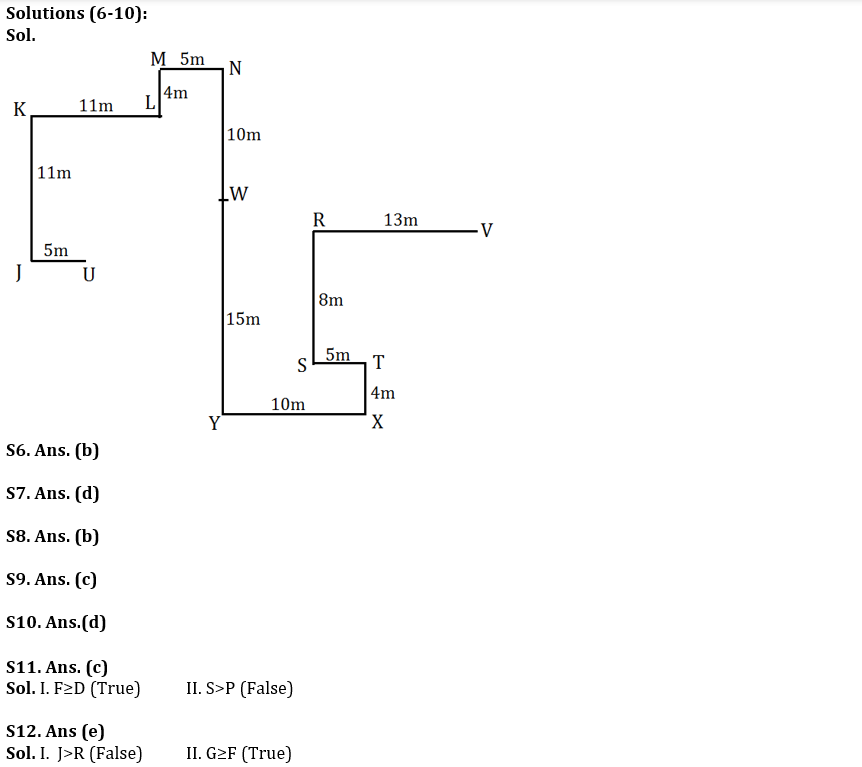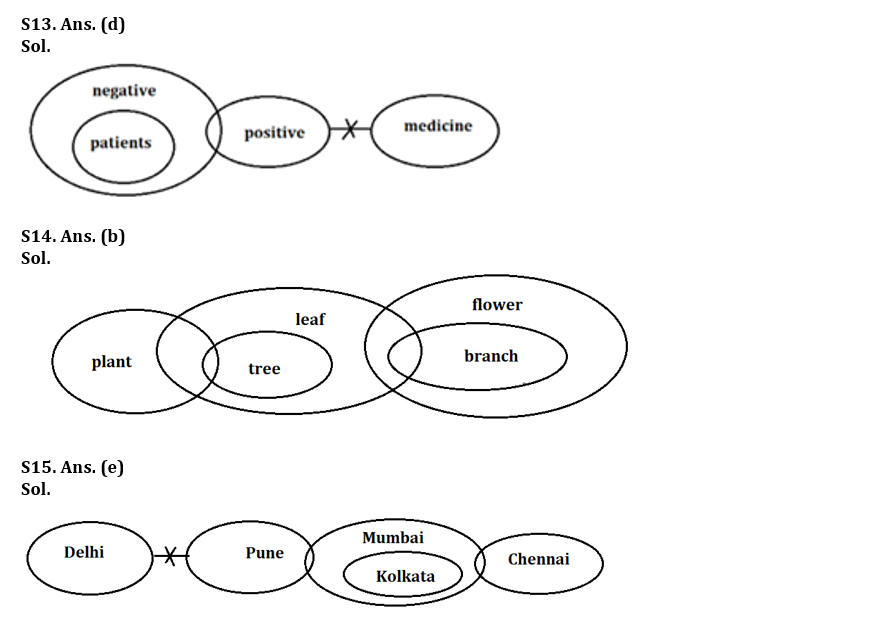Latest Banking jobs   »   Reasoning Ability Quiz For LIC ADO...

# Reasoning Ability Quiz For LIC ADO Mains 2023 – 09th April

Directions (1-5): Study the information carefully and answer the questions given below.
Six persons are sitting around a triangular table, in such a way three persons are sitting three corners facing outside while three are sitting at middle of the side facing inside. Each of them celebrates their birthday on different date 21 to 26 of the same month of the sane year. A sits second to the left of C who is younger than A. Only two persons are sitting between N and B. O is older than B. N is 2days younger than O. M sits second to the right of N. B is not an immediate neighbour of A. Youngest person sits at the middle of the side. B is younger than N but not youngest person. A celebrate his birthday on an odd number date but not on prime number date. Age difference between M and C is 4 days.

Q1. Who among the following celebrate his birthday on 22nd?
(a) C
(b) O
(c) M
(d) N
(e) None of these

Q2. Who among the following person sits second to the right of C?
(a) B
(b) O
(c) M
(d) A
(e) None of these

Q3. How many persons sit between N and M, when counted left of M?
(a) More than three
(b) Two
(c) Three
(d) One
(e) None

Q4. Who among the following is oldest person?
(a) B
(b) O
(c) M
(d) A
(e) None of these

Q5. Who among the following sits third to the right of the second youngest person?
(a) N
(b) C
(c) B
(d) O
(e) None of these

Directions (6-10): Study the following information carefully and answer the questions given below:
P@Q: It means that P is in East of Q
P#Q: It means that P is in West of Q
P\$Q: It means that P is in North of Q
P%Q: It means that P is in South of Q
P(7)\$Q means that P is 7m North of Q
P\$@Q means that P is in North-east of Q

Shubham and Akash are starting their journey from point V and U respectively and both reaches at point W as per the given information-

Shubham- R (13) # V, S (8) % R, T (5) @ S, X (4) % T, Y (10) # X, W (15) \$ Y
Akash- J (5) # U, K (11) \$ J, L (11) @ K, M (4) \$ L, N (5) @ M, W (10) % N

Q6. If point A (2) % R then, what is the direction and shortest distance of A with respect to U?
(a) A (15) \$@ U
(b) A (16) @ U
(c) A (16) \$@ U
(d) A (15) @ U
(e) None of these

Q7. Which of the following is true?
(a) M\$@X
(b) U%@N
(c) U#R
(d) J%#V
(e) None is true

Q8. If B (5) @ L and C (8) # W are true then, what is the shortest distance between B and C?
(a) 5m
(b) 10m
(c) 15m
(d) 20m
(e) None of these

Q9. U is in which direction with respect to V?
(a)North-East
(b)South-East
(c) South West
(d) West
(e) None of these

Q10. What is the direction of T with respect to M?
(a)North-East
(b)South-West
(c) North West
(d) South-East
(e) None of these

Directions (11-12): In the following questions, the symbols %, &, @, \$ and * are used with the following meaning as illustrated below.
‘A % B’ means ‘A is not smaller than B’.
‘A & B’ means ‘A is neither smaller than nor equal to B’.
‘A @B’ means ‘A is neither greater than nor equal to B’.
‘A \$ B’ means ‘A is neither greater than nor smaller than B’.
‘A * B’ means ‘A is not greater than B’.
Now, in each of the following questions assuming the given statements to be true, find which of the four Conclusions I and II given below them is/are definitely true and give your answer accordingly.

Q11. Statements: A&S\$O, P*L&O, D\$A*F
Conclusions: I. F%D II. S&P
(a) Only II is true
(b) Both I and II is true
(c) Only I is true
(d) Either I or II is true
(e) Neither I nor II is true

Q12. Statements: K&F*J\$G&L&O%M@R
Conclusions: I. J&R II. G%F
(a) Only I is true
(b) Neither I nor II is true
(c) Both I and II is true
(d) Either I or II is true
(e) Only II is true

Directions (13-15): In each of the questions below some statements are given followed by conclusions/group of conclusions. You have to assume all the statements to be true even if they seem to be at variance from the commonly known facts and then decide which of the given conclusions logically follow from the information given in the statements:

Q13. Statement: All patients are negative.
Only a few negative is positive.
No positive is medicine.
Conclusions:
(a) All negative can be medicine.
(b) Some medicine can be positive.
(c) No patient is medicine.
(d) Some patients can be positive.
(e) None follows.

Q14. Statement: Only a few plant is tree.
All tree is leaf.
Only a few leaf is branch.
All branch is flower.
Conclusions:
(a) Some flower is tree.
(b) Some plant is leaf.
(c) Some leaf can be flower.
(d) All branch can never be leaf.
(e) All follows.

Q15. Statement: No Delhi is Pune.
Only a few Pune is Mumbai.
Only Mumbai is Kolkata.
Only a few Mumbai is Chennai.
Conclusions:
(a) Some Chennai can be Kolkata.
(b) Some Pune can be Kolkata.
(c) Some Delhi is not Mumbai.
(d) All Mumbai can be Pune.
(e) None follows.

Solutions## FAQs

### When is the LIC ADO Mains Exam scheduled?

The LIC ADO Mains exam is scheduled to be held on 23 April 2023

#### Congratulations!Union Budget 2023-24: Free PDF